• 大可以理解为该维度上尺子的单位刻度大，比如表示一个单位刻度 小可以理解为该维度上尺子的单位刻度小，比如表示一个单位刻度 (二) 医学上 如果把矩阵理解为中医祖传秘籍(乱不外传的)，特征向量理解为秘方子(枸杞、...毕业多年，曾经有同事问我该如何理解特征值的意义？

当时，实在羞愧，我一学数学的，真不知该如何回答。

极力回想，也只能以“特征值的求法、步骤...bla...bla...”应付了事，

答非所问，简直了得！

人家问的是意义，如何理解其意义？

直扣灵魂，

我真的曾经理解过它的意义吗？？？

招了吧，真没有！

原在数学系时，教室里，对着黑板一堆密密麻麻的公式，我也是时常神游天外的主......

考试前，为了避免挂科才熬夜突击，对着书本一一比划，至少要演算两到三张稿纸，才能勉强记住方法、步骤，哪还管得着它的意义？

这种突击式的训练记忆，忘得也快，就像写代码一样，过一阵就忘了！

课堂上，老师大多是照本宣科。

当年，

也许是自己知识阅历不够，很难理解其意义，

也许是努力不够，被足球耽误了。

也许是天赋所致，不能顿悟！

...

总之，确定那时我肯定是没有理解它的意义的。

不知道现在有多少学生还是一样？

在学习一些抽象的数学工具时，代换三、四步之后就不知所云了，往往只能靠记忆强撑，而这种记忆最多维持一周，年轻时可能长点，后来，说忘就忘了......。

有极少数天才，能在抽象世界里面一直转，抽啊抽，一直抽......并最终以此为业。

而大多数人(99+%)，一到毕业，就尴尬，因为真的不理解其意义，

看似学了一些高深的数学知识，只会做题，不会运用，根本不理解公式指代符号的现实映射！进而职场上，其它方面训练缺失的短板逐渐显现后，囧是必然！

我想，这不单是数学教育的问题，也是其它各方面可能会尴尬的本源：

不理解意义

好，扯远了，回到正题，来看灵魂之问：

如何理解特征值的意义？

最近才有些感悟，和大家分享一下。

说到特征值，数学上，基本上是指矩阵的特征值

说到矩阵，高等代数几乎一整本书都在讲它，最著名的数学软件叫Matlab，直译为矩阵实验室，足见其高深、复杂！

而这么复杂混乱的东西确有一个特征值， 难道不奇怪？

再说，矩阵到底有多复杂混乱？看数学公式体会一下吧：

这是一堆数，每一个数字都可以在实数域内取值(正、负、零)，或可以无限的延伸，联想到现在的大数据，还有什么东西不能由它表示呢？如果您相信万物皆数，这儿都可以说万物皆矩阵了，万物，能不复杂？

另外，这一堆数既可以表示数据记录，还可以表示某种不知名的抽象运算(物理上叫算子)，这样的数学运算，对某些对象集，确仅仅以固有的方式伸缩，且不管它是数据记录还是抽象运算，全都一样！

如此混乱复杂！ 确有本征!

这不神奇吗？

数学就是这样，抽象、高级、有理

如果这样说感觉虚，那么先来看一下它精确(枯燥)的数学定义

特征值

设是一矩阵，是一维非零列向量，若存在一数，使得

则称为的一个特征值，为的属于特征值的一个特征向量。

展开

， 即

若把矩阵的每一行理解为一个基向量，则是表示基向量与该向量的内积() 等于。

感觉公式真的很枯燥的话，就先跳过上面吧。

下面我将从三个方面来试图阐释其意义，以便大家更好的理解。

• 几何上
• 医学上
• 物理上

(一)几何上

如果把矩阵理解为一个坐标系(不一定直角的，也可能是严重变形的“尺子”)，有一类向量叫特征向量，其中的任一个向量，在该矩阵上的投影，都只是自身固定的伸缩！

如何理解投影呢？且拿三维来理解吧，一根射线在另外一个坐标系(矩阵)下的影子，其每一轴都会有投影分量，把所有分量组合还原成影子，跟其自身共线，影子射线的长度比值永远固定，这个比值就是特征值，简如下图。

而该比值对这条直线上的所有向量都适应，即无论射线长短。那么有多少条这样的直线呢？维矩阵最多有条，每一条的比值(特征值)可能都不一样，有大有小，都代表这一维度的自身特征，故这里大、小意义就明显了。

• 大可以理解为该维度上尺子的单位刻度大，比如表示一个单位刻度
• 小可以理解为该维度上尺子的单位刻度小，比如表示一个单位刻度

(二) 医学上

如果把矩阵理解为中医祖传秘籍(乱不外传的)，特征向量理解为秘方子(枸杞、百合、红花、童子尿...)，特征值就是对该方子的用药量，温、热、寒不同方子特征值不一样， 这样也说得通，如下图！

进一步，把西药制成品也类比为特征向量。比如新冠治疗中的瑞得西韦， 特征值就是该神药该服用多少？还有其它药方子，如莲花清瘟等，假设都能治疗新冠肺炎，但用量肯定是不一样的，即不同特征向量对应的特征值不一。

如此看来，特征值可理解为医学上药物用量的一个刻度，也是中西医互相密而不宣的沟通桥梁，正如下图的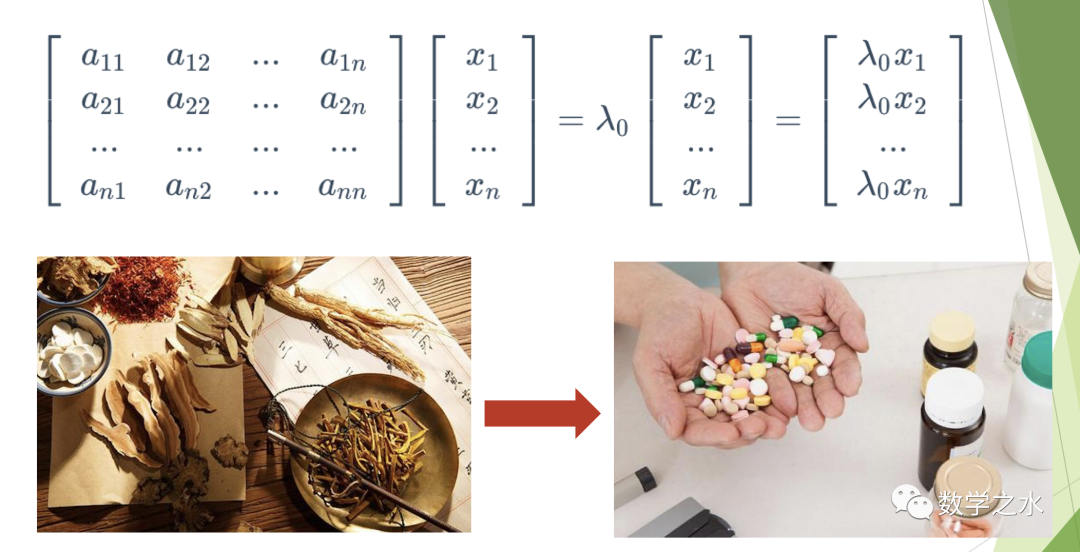(三) 物理上

“遇事不决，量子力学” 戏谑的表明了量子力学的高深、难懂！

且看薛定谔方程的前半部分，就复杂得都让人头晕眼花.....

物理学家把这种神操作统称为算子(因为给您解释不清楚~)，是不是有点巫师作法、道士占卜的感觉？

不同的是那帮巫师(物理学家)，在圈内对不同公式符号都给出了互相认可的解释！

例如：量子力学把世界看成是波动的，如果一个波函数经过一个量子变换后，它仍是一同一个波函数乘一个常量(如上图C)。

......

再看矩阵，它不也就是一个算子吗？而且还是线性的，如此简单，so easy!

大巫师(物理学家)牛！

这样，特征值的意义又从矩阵的线性上升到非线性统一了。

还是大巫师(物理学家)牛~

总之，就是一段复杂的操作，统称为算子特征值也叫算子的本征值，台湾人习惯这样称呼，同一个意思，英文词源其实来自德语(自身的)。本来很好理解的概念，几经"转手"之后就晦涩难懂了....

遥想当年，若彼时能有这样的理解，就完美了！

想记这点感悟也很久了，

若有缘遇上，能给您带来一点点共鸣，便是满足最后附上特征值的求法，以便大家回忆。

附：特征值求法

特征多项式

它是数域上的一个次多项式，若是复数域，必有个根。每一个根都是矩阵的一个特征值

求特征值与特征向量方法步骤

• 求出特征多项式的全部根
• 把所求根代入方程组中求出一组基础解系，就得到属于相应特征值的线性无关的特征向量

数学之水，更多免费干货、敬请关注详查~~展开全文复数特征值求特征向量
• 理解复数域上的向量空间

千次阅读 2021-04-05 10:00:26
说明：本文转载自博客：理解复数域上的向量空间（第一篇），由于没有找到原作者的联系方式，所以直接搬运过来了，如有侵权请联系我，我立即删除。 线性代数进行到酉空间中的自伴算子、正规算子以及谱定理这部分内容...

说明：本文转载自博客：理解复数域上的向量空间（第一篇），由于没有找到原作者的联系方式，所以直接搬运过来了，如有侵权请联系我，我立即删除。

线性代数进行到酉空间中的自伴算子、正规算子以及谱定理这部分内容时，会发现很多在复空间中成立的命题在实空间中却未必成立。这种情况多少让人感到有点奇怪，为什么会出现这种情况？
复数域是包含实数域的，我们学习复数之后碰到最多的是相反的情况：原本在实数域上成立的性质在复数域中不一定成立了，比如，实数可以比较大小，但复数没有大小关系；又比如，实数的平方非负，等等。这样的命题见多了，容易使人产生思维定势，认为复数包含实数，因此在复数范围内成立的命题在实数范围内也必然成立，而实数范围成立的命题不一定都能推广到复数。
可尤其是学习到复变函数之后，这种情况似乎反过来了，同样的一个概念，到了复数中反倒比原来实数情况下的相应概念有了更多的内涵。这又是为什么呢？

比如，在”Linear Algebra Done Right” 第七章有个命题 7.2，是说

命题7.2： 如果 V V 是复数域上的内积空间，并且 T T V V 上的线性算子，且对任意向量 v v ，都有 ⟨ T v , v ⟩ = 0 \langle Tv,v\rangle=0 ，那么 T = 0 T=0
证明： 使用恒等式
⟨ T u , w ⟩ = ⟨ T ( u + w ) , u + w ⟩ − ⟨ T ( u − w ) , u − w ⟩ 4 + ⟨ T ( u + i w ) , u + i w ⟩ + ⟨ T ( u − i w ) , u − i w ⟩ 4 i \begin{aligned}\langle Tu,w\rangle=&\frac{\langle T(u+w),u+w\rangle-\langle T(u-w),u-w\rangle}{4}\\ &+\frac{\langle T(u+iw),u+iw\rangle+\langle T(u-iw),u-iw\rangle}{4}i\end{aligned}
即可得证。

但是，同样的假设，在实数空间中却得不出同样的结论来，比如，二维空间中把所有向量都逆时针旋转90度角。

可是，在实空间中可以存在旋转90度的映射，为什么在复空间中就没有这种映射？难道就不可以有一个线性变换像实空间中那样把每一个向量都旋转到垂直的位置上吗？

它的证明的确在那里，证法也的确没有错，但是我却从直观上难以接受这个命题。我不甘心，于是找来实空间中的那个旋转映射放在复空间上，定义复空间上的映射
T ( a b ) = ( b − a ) T\begin{pmatrix}a\\ b\end{pmatrix}=\begin{pmatrix}b\\ -a\end{pmatrix}
那么 ⟨ T v , v ⟩ = a b ˉ − b a ˉ \langle Tv,v\rangle=a\bar b-b\bar a ，通常情况下，这是个纯虚数，不一定是零。所以这个反例失败了，在这个例子上，我不得不承认上面那个命题。但是，它给我带来更多的思考：

我们应该怎样直观地想象复向量空间？从前我们总是把向量想象成一条带有方向的线段，把一维子空间想象成一条直线，”线”总是伴随着我们对向量空间的影像理解，因为实数上面可以定义线序，而我们生存的三维空间就可以看成实三维空间，所以在理解一般的向量空间的时候，这种影像可以帮助我们建立起很多问题的直观。但是，当探究复向量空间内部的特殊结构的时候，这种形象的理解遇到了一些问题。
比如，把一个复向量取共轭，跟原来的向量是什么位置关系？又比如，一个向量 v v 乘以 i i ，变成了 i v iv ，是把这个向量怎么样了？旋转了九十度？可是毕竟二者是线性相关的，而在我们的直观理解中，线性相关的两个向量是共线的，数乘只是把向量拉长或缩短。但是如果把它们两个想象成共线的，又无法想象它们两个有相同的长度，一个一维子空间中相同长度的向量不是两个，而是无穷多个，无法想象。
看来，与复平面类似，只有把一维复向量空间理解成我们所熟悉的平面才自然一些，而这个一维复空间中的一个复向量，可以看成是躺在个平面上的，它并不占据这个一维空间的一整段，在它周围可以有无数多条与它长度相等的向量。
那么这么看来，一个 n 维的复空间，是否可以形象地把它想象成一个 2n 维实空间？

把问题提得更明确一些，如果我们把一个 n 维复空间看成一个实数域上的向量空间，即把数量乘法中用到的数限制在实数域中，那么，原来的基底 e 1 , e 2 , … , e n e_1,e_2,\dots,e_n 就张不成整个空间了，可以证明， 向量组 e 1 , i e 1 , e 2 , i e 2 , … , e n , i e n e_1,ie_1,e_2,ie_2,\dots,e_n,ie_n 是这个实向量空间的基底，一共 2n 个元素，所以它是一个 2n 维实向量空间。（注意，这个实数空间中的一个向量 v 在原来复空间意义下可以乘以纯量 i 变成 iv，但在实空间中 iv 将不再是这种乘法的结果，它只是与 v 有关的一个向量而已。我们仍然记为 iv。）

如果只考虑空间本身，那么这样的理解是足够的，也足以提供复空间的一些直观信息。可是，我们需要理解的不止是这些，还有内积、线性变换等一些东西，在这个衍生出来的实空间中的这些东西到底跟原来的复空间中相应的对象有什么联系？

首先分析内积，如果原来的复空间中有内积 ⟨ ⋅ , ⋅ ⟩ \langle \cdot,\cdot\rangle ，那么 R e   ⟨ ⋅ , ⋅ ⟩ \mathrm{Re}\,\langle \cdot,\cdot\rangle 就是衍生出的实空间中的内积，我们用方括号 [ ⋅ , ⋅ ] [\cdot,\cdot] 表示这个实空间的内积，
那么原来的内积就可以用这个实数空间内积表示为
⟨ u , v ⟩ = [ u , v ] + i [ u , i v ] \langle u,v\rangle=[u,v]+i[u,iv]
并且，这两个内积诱导的范数是相同的。
对任意向量 v v ，向量 i v iv 在实数空间的内积意义下是垂直于 v v 的： [ i v , v ] = R e   i ⟨ v , v ⟩ = 0 [iv,v]=\mathrm{Re}\,i\langle v,v\rangle=0
如果 e 1 , e 2 , … , e n e_1,e_2,\dots,e_n 是复空间的标准正交基底，那么 e 1 , i e 1 , e 2 , i e 2 , … , e n , i e n e_1,ie_1,e_2,ie_2,\dots,e_n,ie_n 是实空间的标准正交基底。

下面讨论一个向量乘以 i 是怎么回事。在复空间中，变换 S = i I S=iI 是个线性变换，那么在实空间中 v v i v iv 的变换是否也是线性变换？注意到原来复空间的任何线性变换在实空间下也是线性变换，所以 S S 也不例外。那么它在实空间意义下对应什么矩阵呢？
取上面的标准正交基底 e 1 , i e 1 , e 2 , i e 2 , … , e n , i e n e_1,ie_1,e_2,ie_2,\dots,e_n,ie_n ，显然它在这组基底下的矩阵为
( 0 − 1 0 0 1 0 0 0 ⋱ 0 0 0 − 1 0 0 1 0 ) \begin{pmatrix}0&-1&&0&0\\ 1&0&&0&0\\ &&\ddots&&\\ 0&0&&0&-1\\ 0&0&&1&0\end{pmatrix}
即主对角线上是 2×2 阶矩阵块
( 0 − 1 1 0 ) \begin{pmatrix}0&-1\\ 1&0\end{pmatrix}
其余位置都为零。

接下来讨论一般的线性变换。在复空间中每个线性变换 T T 都对应实空间的一个线性变换，那么反过来，是否一个实空间的线性变换在复空间中也是线性变换呢？
T ( u + v ) = T u + T v T(u+v)=Tu+Tv 这一条是两种空间都共同满足的，但关键是 T ( k v ) = k T v T(kv)=kTv 这个性质，对于实空间，我们只要求 k k 为实数，但对于复空间，我们还要求 k k 可以取复数。那么就必须有 T ( a u + b i u ) = a T u + b i T u T(au+biu)=aTu+biTu ，换句话说，就是 T T 与上面定义的 S S 可交换： T S v = S T v TSv=STv ，只有这样的 T T 才有资格作为复空间的线性变换，并且这是个充要条件。
分析一下 L ( V ) \mathcal L(V) 的维数，当 V V 是复空间时， L ( V ) \mathcal L(V) 的维数是 n 2 n^2 ，把 L ( V ) \mathcal L(V) 看成相应的实数空间，维数也不过是 2 n 2 2n^2 ，但如果把 V V 看成相应的实空间，那么 L ( V ) \mathcal L(V) 的维数是 4 n 2 4n^2 ，可见，复空间上的线性变换比相应的实空间的线性变换少了一半，原因就是复空间的特性对线性变换会有更多的限制。
利用矩阵的分块乘法规则可以证明，实空间上的 2 n × 2 n 2n\times 2n 阶矩阵 A A ，如果与 S S 对应的矩阵可交换，那么 A A 有以下形式：
( a − b c − d b a d c ⋱ e − f g − h f e h g ) \begin{pmatrix}a&-b&&c&-d\\ b&a&&d&c\\ &&\ddots&&\\ e&-f&&g&-h\\ f&e&&h&g\end{pmatrix}
显然，它对应复空间上的矩阵
( a + b i … c + d i ⋮ ⋱ ⋮ e + f i … g + h i ) \begin{pmatrix}a+bi&\dots&c+di\\ \vdots&\ddots&\vdots\\ e+fi&\dots&g+hi\end{pmatrix}
而且，分析这种矩阵的自由度，与上面分析的复空间变换的维数恰好吻合。

下面利用实空间的理论，在复空间衍生的实空间中证明命题 7.2。首先证明一条引理：

引理1： 如果 V V 是实的内积空间， T ∈ L ( V ) T\in\mathcal L(V) ，那么 T T 满足
∀ v ∈ V , ⟨ T v , v ⟩ = 0 \forall v\in V,\langle Tv,v\rangle=0
当且仅当 T T 是斜自伴的，即 T ⋆ = − T T^\star=-T
证明： 如果 T ⋆ = − T T^\star=-T ，那么有 ⟨ T v , v ⟩ = ⟨ v , T ⋆ v ⟩ = − ⟨ v , T v ⟩ = − ⟨ T v , v ⟩ \langle Tv,v\rangle=\langle v,T^\star v\rangle=-\langle v,Tv\rangle=-\langle Tv,v\rangle ，因此 ⟨ T v , v ⟩ = 0 \langle Tv,v\rangle=0
反过来，如果 ∀ v ∈ V , ⟨ T v , v ⟩ = 0 \forall v\in V,\langle Tv,v\rangle=0 ，那么
⟨ T ( v + w ) , v + w ⟩ = ⟨ T v , v ⟩ + ⟨ T v , w ⟩ + ⟨ T w , v ⟩ + ⟨ T w , w ⟩ = 0 \begin{aligned}\langle T(v+w),v+w\rangle=&\langle Tv,v\rangle+\langle Tv,w\rangle+\langle Tw,v\rangle+\langle Tw,w\rangle\\ =&0\end{aligned}
因此
⟨ T v , w ⟩ + ⟨ T w , v ⟩ = ⟨ T v , w ⟩ + ⟨ w , T ∗ v ⟩ = ⟨ T v , w ⟩ + ⟨ T ∗ v , w ⟩ = ⟨ ( T + T ∗ ) v , w ⟩ = 0 \begin{aligned}\langle Tv,w\rangle+\langle Tw,v\rangle=&\langle Tv,w\rangle+\langle w,T^*v\rangle\\ =&\langle Tv,w\rangle+\langle T^*v,w\rangle\\ =&\langle (T+T^*)v,w\rangle\\ =&0\end{aligned}
w = ( T + T ∗ ) v w=(T+T^*)v 即可得证。

接下来把命题7.2 翻译成实空间中的命题并证明之。

命题2 V V 是实内积空间， T T S S 都是 V V 上的斜自伴算子，且 S S 可逆， T T S S 可交换，并且任意向量 v v ⟨ T v , S v ⟩ = 0 \langle Tv,Sv\rangle=0 ，那么 T = 0 T=0
证明：由 ⟨ T v , S v ⟩ = − ⟨ S T v , v ⟩ = 0 \langle Tv,Sv\rangle=-\langle STv,v\rangle=0 ，可知 S T ST 也是斜自伴的。那么
− T S = ( T S ) ∗ = S ∗ T ∗ = S T = T S -TS=(TS)^*=S^*T^*=ST=TS ，因此 T S = 0 TS=0 。又 S S 可逆，故 T = 0 T=0 。证毕。
（P.S: 如果没有 S S 可逆的条件，那么 n u l l   T ⊃ r a n g e   S \mathrm{null}\,T\supset\mathrm{range}\,S 。）

原来一个复向量空间上的线性变换相当于暗中假定了这么多的条件！难怪复空间中的算子理论有那么好的性质。

2015/10/28 补充：

我们可以完善引理1，使得通过这个引理可以更直接地证明书上的命题7.2：

引理1‘： V V 是实数域或复数域上的内积空间， T ∈ L ( V ) T\in\mathcal L(V) 。记 i R i\mathbb R 为所有纯虚数和 0 0 构成的集合，那么， T T 满足
∀ v ∈ V , ⟨ T v , v ⟩ ∈ i R \forall v\in V,\langle Tv,v\rangle\in i\mathbb R
当且仅当 T T 是斜自伴的，即 T ∗ = − T T^*=-T
证明： 如果 T ∗ = − T T^*=-T ，那么有 ⟨ T v , v ⟩ = ⟨ v , T ∗ v ⟩ = − ⟨ v , T v ⟩ = − ⟨ T v , v ⟩ ‾ \langle Tv,v\rangle=\langle v,T^*v\rangle=-\langle v,Tv\rangle=-\overline{\langle Tv,v\rangle} ，因此 ⟨ T v , v ⟩ ∈ i R \langle Tv,v\rangle\in i\mathbb R
反过来，如果 ∀ v ∈ V , ⟨ T v , v ⟩ ∈ i R \forall v\in V,\langle Tv,v\rangle\in i\mathbb R ，那么
⟨ T ( v + w ) , v + w ⟩ = ⟨ T v , v ⟩ + ⟨ T v , w ⟩ + ⟨ T w , v ⟩ + ⟨ T w , w ⟩ ∈ i R \begin{aligned}\langle T(v+w),v+w\rangle=&\langle Tv,v\rangle+\langle Tv,w\rangle+\langle Tw,v\rangle+\langle Tw,w\rangle\\ \in& i\mathbb R\end{aligned}
因此
ℜ ⟨ T v , w ⟩ + ⟨ T w , v ⟩ = ℜ ( ⟨ T v , w ⟩ + ⟨ w , T ∗ v ⟩ ) = ℜ ( ⟨ T v , w ⟩ + ⟨ T ∗ v , w ⟩ ) = ℜ ⟨ ( T + T ∗ ) v , w ⟩ = 0 \begin{aligned}\Re\langle Tv,w\rangle+\langle Tw,v\rangle=&\Re(\langle Tv,w\rangle+\langle w,T^*v\rangle)\\ =&\Re(\langle Tv,w\rangle+\langle T^*v,w\rangle)\\ =&\Re\langle (T+T^*)v,w\rangle\\ =&0\end{aligned}
w = ( T + T ⋆ ) v w=(T+T^\star)v 即可得 ∀ v , ∥ ( T + T ⋆ ) v ∥ 2 = 0 \forall v, \|(T+T^\star)v\|^2=0 ，因此 T = − T ⋆ T=-T^\star

这样，在复数域空间中，如果 T T 满足 ∀ v ∈ V , ⟨ T v , v ⟩ = 0 \forall v\in V,\langle Tv,v\rangle =0 ，那么 T T i T iT 就同时满足引理1’的条件，所以同时有 T = − T ⋆ T=-T^\star i T = − ( i T ) ⋆ = i T ⋆ iT=-(iT)^\star=iT^\star ，因此 T = 0 T=0

展开全文线性代数 向量空间
• 那篇文章是在2月份完成的，那时还没有系统地学习向量复数的相关知识，现在，BoJone从向量复数两个角度，给出关于旋转公式的两个证明，仅供参考，如有错误，请指出。为了把问题化简，我们先做以下平移：...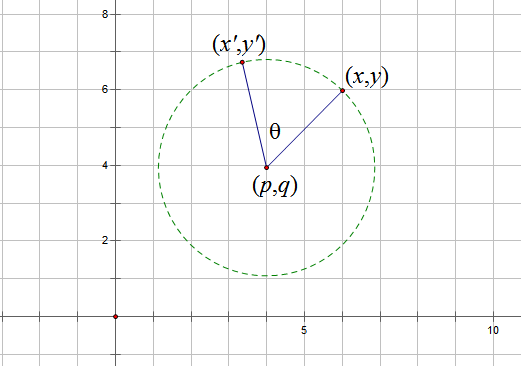坐标旋转

如图，坐标(x,y)绕点(p,q)逆时针旋转θ角后得到坐标(x',y')，求x',y'关于x,y的表达式。

之前我们已经讨论过这个问题，在《函数图像旋转公式》一文中，利用解析几何的方法进行了分析。那篇文章是在2月份完成的，那时还没有系统地学习向量和复数的相关知识，现在，BoJone从向量和复数两个角度，给出关于旋转公式的两个证明，仅供参考，如有错误，请指出。

为了把问题化简，我们先做以下平移：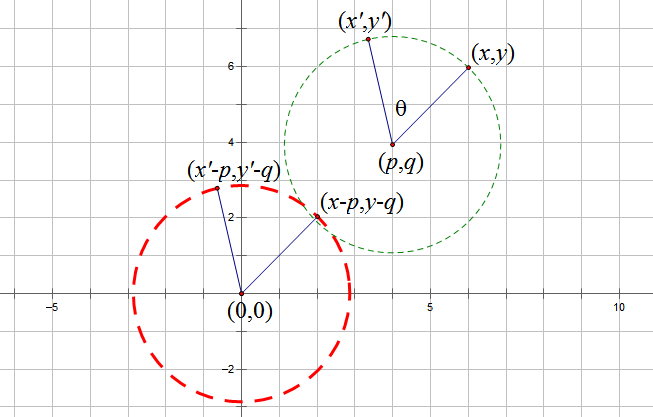坐标旋转-平移

这样我们只需讨论旋转中心位于原点的问题。首先我们利用向量来求解。旋转前后的两个点分别用向量表示为$\vec{A}=(x-p,y-q,0),\vec{B}=(x'-p,y'-q,0),|\vec{A}|=|\vec{B}|=R$，那么有$\vec{A}\times\vec{B}=(0,0,(x-p)(y'-q)-(x'-p)(y-q))$，并且

$$\vec{A}\cdot \vec{B}=R^2 \cos\theta=(x-p)(x'-p)+(y-q)(y'-q)\tag{1}$$$$|\vec{A}\times\vec{B}|=|R^2 \sin\theta|=|(x-p)(y'-q)-(x'-p)(y-q)|\tag{2}$$

考虑$0 < \theta$(1)\times (y-q)+(2)\times (x-p)得到 \begin{aligned}(y'-q)[(y-q)^2+(x-p)^2]=R^2[(y-q)\cos\theta+(x-p)\sin\theta] \\ y'-q=(y-q)\cos\theta+(x-p)\sin\theta\end{aligned}\tag{3}(1)\times (x-p)+(2)\times (y-q)得到 \begin{aligned}(x'-p)[(y-q)^2+(x-p)^2]=R^2[(x-p)\cos\theta-(y-q)\sin\theta] \\ x'-p=(x-p)\cos\theta-(y-q)\sin\theta\end{aligned}\tag{4} (3)和(4)就是坐标旋转公式。在\pi < \theta <2\pi$时形式一样。 接下来使用复数解答。我们知道，复数可以用复平面表示，并且两个复数相乘，结果也是复数，其模等于乘数模的积，辐角等于乘数辐角的和。于是我们不妨用$z_1=(x-p)+(y-q)i$来表示旋转前的点，用$z_2=(x'-p)+(y'-q)i$表示旋转后的点。很明显，z2是由z1乘上一个模等于1、辐角为θ的复数，不难得出，这个复数就是$cos\theta+i*sin\theta\$。也就是说

\begin{aligned}[(x-p)+(y-q)i]\cdot [\cos\theta+(\sin\theta)i]=(x'-p)+(y'-q)i \\ [(x-p)\cos\theta-(y-q)\sin\theta]+[(y-q)\cos\theta+(x-p)\sin\theta]i \\ =(x'-p)+(y'-q)i\end{aligned}

根据复数相等的条件就有

\begin{aligned}y'-q=(y-q)\cos\theta+(x-p)\sin\theta \\ x'-p=(x-p)\cos\theta-(y-q)\sin\theta\end{aligned}

利用复数解答几何问题很重要的一点就是应用复数相等的条件是“实数部分=实数部分，虚数部分=虚数部分”。这样有时可以把问题回归到实数的范畴内，进而利用已知的知识解答。要想把复数更好地应用于几何，还要熟悉复平面的应用，关键是理解复数相关运算的几何意义。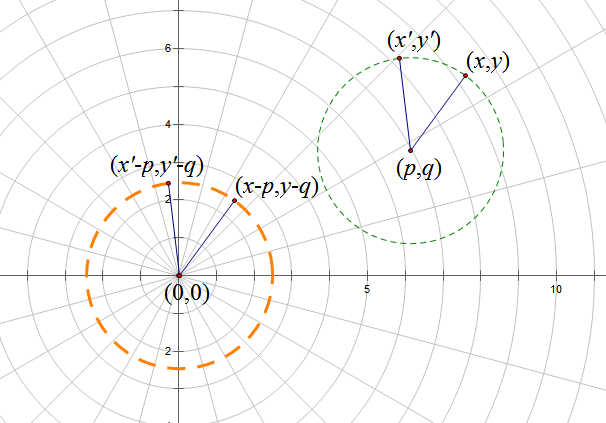坐标旋转-极坐标

最后看一个利用极坐标的推导，由上图可以看出

$$x-p=r \cos f,y-q=r \sin f,r=\sqrt{(x-p)^2+(y-q)^2}$$

并且有

\begin{aligned}x'-p= r \cos (f+\theta)=r \cos f \cos\theta-r \sin f \sin\theta=(x-p)\cos\theta-(y-q)\sin\theta \\ y'-q= r \sin (f+\theta)=r \sin f \cos\theta + r \cos f \sin\theta=(y-q)\cos\theta+(x-p)\sin\theta\end{aligned}

证毕。

更详细的转载事宜请参考：《科学空间FAQ》

如果您还有什么疑惑或建议，欢迎在下方评论区继续讨论。

如果您觉得本文还不错，欢迎分享/打赏本文。打赏并非要从中获得收益，而是希望知道科学空间获得了多少读者的真心关注。当然，如果你无视它，也不会影响你的阅读。再次表示欢迎和感谢！

打赏

微信打赏

支付宝打赏

因为网站后台对打赏并无记录，因此欢迎在打赏时候备注留言。你还可以点击这里或在下方评论区留言来告知你的建议或需求。

如果您需要引用本文，请参考：

展开全文• 在理解复数和虚数之前我们先看如何表示一个笛卡尔积坐标系下过原点的向量，如下图一所示 图一 向量之间还可以通过加法来合成，如下图二所示 图二 除此之外向量之间还有点乘和叉乘，但唯独没有向量乘法，即:...

原创文章，转载请注明出处：https://blog.csdn.net/weixin_37864449/article/details/103854299

在理解复数和虚数之前我们先看如何表示一个笛卡尔积坐标系下过原点的向量，如下图一所示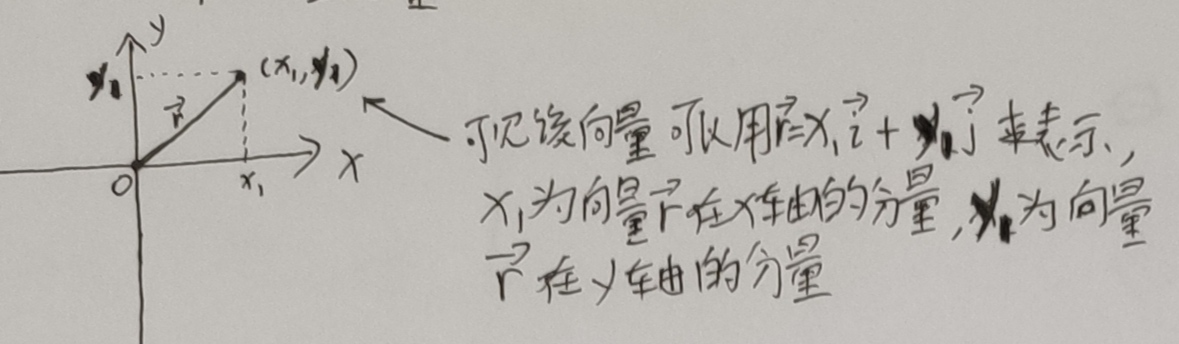图一

向量之间还可以通过加法来合成，如下图二所示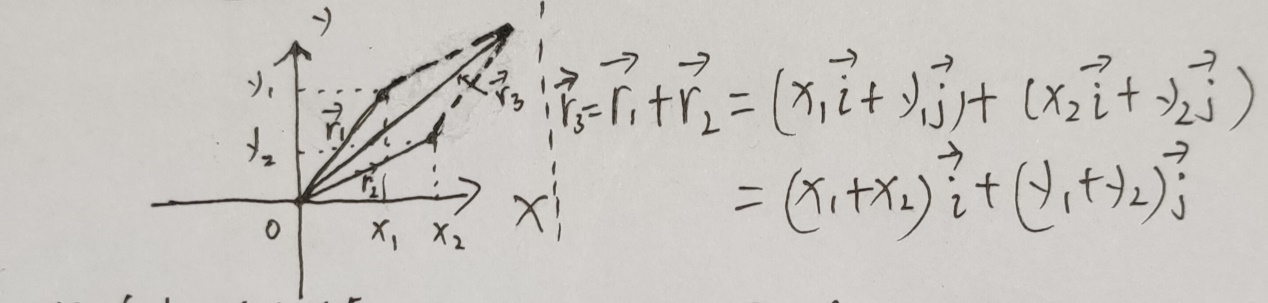图二

除此之外向量之间还有点乘和叉乘，但唯独没有向量乘法，即: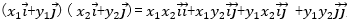。另外向量加法，点乘和叉乘运算都不能控制向量旋转，为此人们就想到了是否可以使用还没利用到的向量乘法来表示向量旋转。显然要实现向量旋转的目的，就需要使用新的模型表示过原点的向量，为此诞生了复数。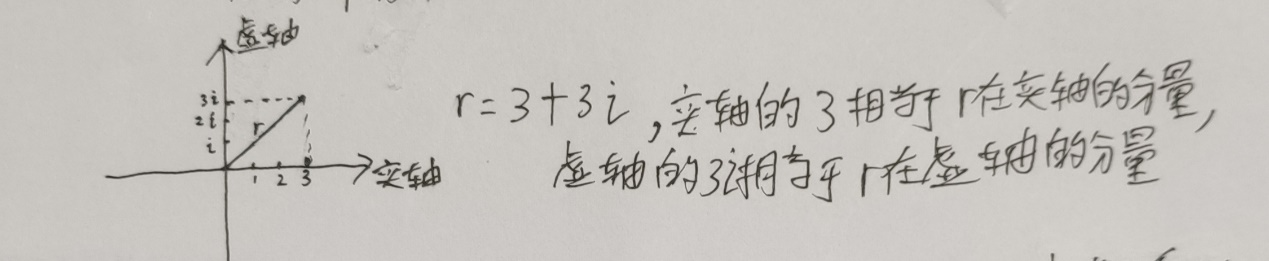图三

如图三所示就是用复数表示向量的模型。同理复数加法运算(a+bi)+(c+di)=(a+c)+(b+d)i相当于向量的加法合成，合成的向量还是用复数表示。下面为了使复数表示的向量乘法能表示向量的旋转，我们制定了如下用乘法表示的旋转规则，见图四：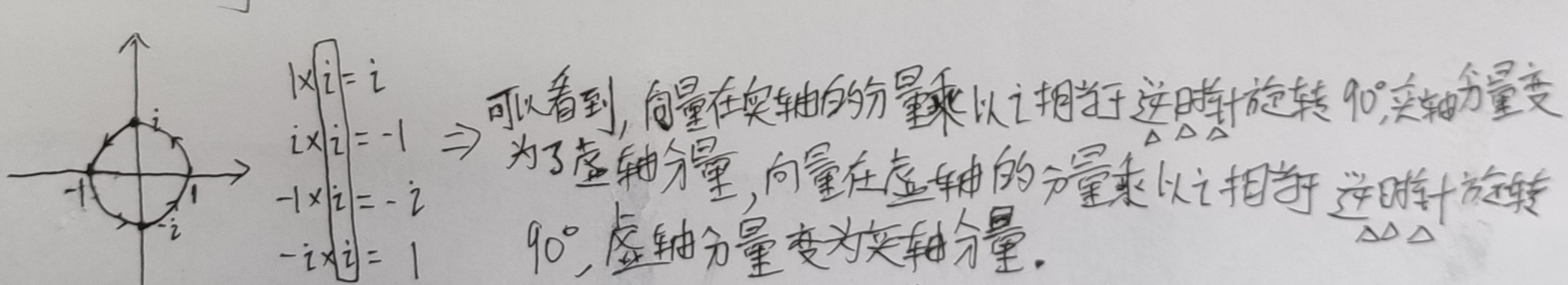图四

我们知道，要控制向量旋转，实质上就是控制向量在实轴的分量和虚轴分量的大小，由上图四乘法旋转规则可知，实轴分量大小可以由虚轴分量大小乘以 i 控制，而虚轴分量大小又可以用实轴分量大小乘以 i 控制，这样就开始有了交叉相乘的味道。

在介绍复数表示的向量乘法之前先介绍复数模和幅角的概念，为了描述复平面上的任意一点（即过复平面原点的向量），可以写成更为普遍的形式：

z=a+bi

其中a和b分别称为复数Z的实部虚部。而Z的长度或“模(Modulus)”为Z点到复平面圆心处的距离,即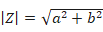,Z的幅角公式为下图五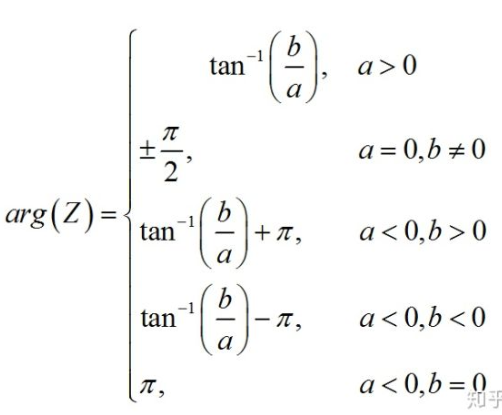图五(注: 幅角即为向量与实轴的夹角)

现在我们开始介绍重头戏：复数表示的向量乘法，假设r1=a+bi,r2=c+di,则

下面引用知乎"怎么理解复数和虚数的一个例题说明复数乘法的应用：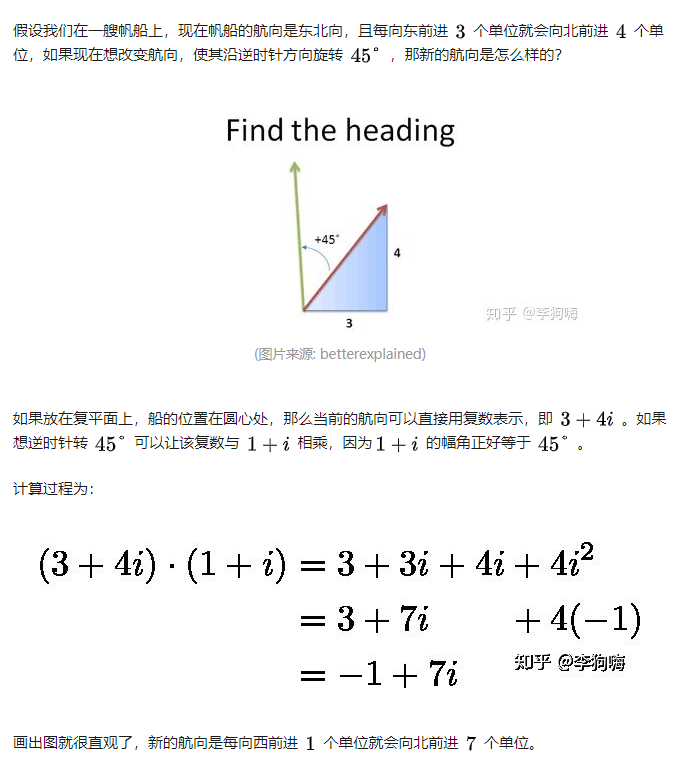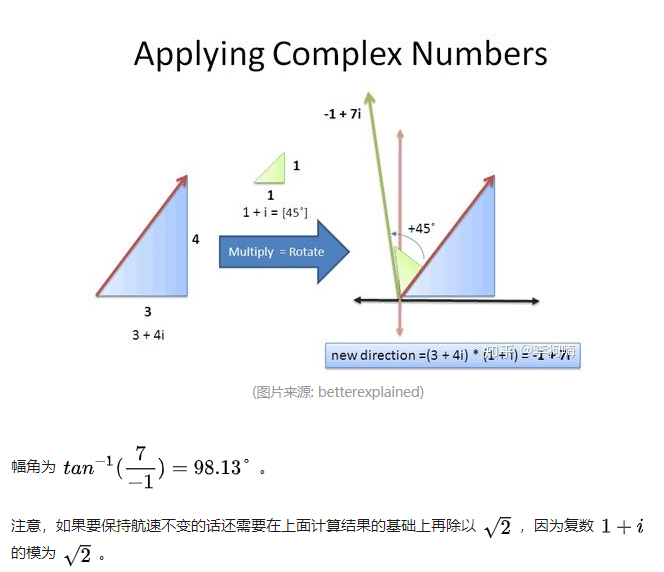展开全文数学 复数 虚数 向量
• matlab复数表示

2021-04-22 02:17:24
第三章:MATLAB 的基础知识(基本符号,数据类型,运算符, 复数运算,三角函...MATLAB 常用的基本数学函数 abs(x):纯量的绝对值或向量的长度 angle(z):复数 z 的相角(Phase angle) sqrt(x):开平方 real(z):复数 z 的实部 ...
• 1、两点坐标表示方向向量 2、向量法计算点到直线的距离 上面2张图来源于 这里 3、C++ norm() 可以返回复数的平方大小 4、向量a与b叉乘，可以得到向量a与b组成的平行四边形的面积，平行四边形的面积又等于...
• 二：正弦量的向量表示法 瞬时值小写 有效值：大写 幅值：大写+m 向量：大写+点 向量的表达方式：U一点=U的有效值，角，初相位 实质是复数表示正弦量 设A为复数，则有： 综：一个复数可以表示成四种形式： 代数式，...
• 正弦量的向量表示

千次阅读 2020-05-24 16:48:28
实质：用复数表示正弦量 复数表示形式 设A为复数; 代数式 三角式 指数式 极坐标式 复数的模即为正弦量的幅值(或有效值) 复数的辐角即为正弦量的初相角 注意： 相量只是表示正弦量，而不等于正弦量，两者...
• 下面将介绍一下正弦量可以用向量表示的原理，也提一下复数、极坐标等知识，做个人学习之用。 一，正弦量用向量表示的原理计算。 假设对于任意一个正弦量：Asin⁡aA\sin aAsina，我们都可以在坐标轴上将它表示出来： ...
• 【新教材】7.3.1 复数的三角表示式教学设计(人教A版)教材分析《复数的三角形式》是复数这一章中的一个重要内容，引进复数三角式的依据是复数的几何意义和三角函数的定义，它是数形结合的产物，有了它就可借助三角...
• 词的向量表示

万次阅读 2014-04-30 12:59:13
（不知道这个应该怎么翻译，因为还存在一种叫“Distributional Representation”的表示方法，又是另一个不同的概念）表示的一种低维实数向量。这种向量一般长成这个样子：[0.792, −0.177, −0.107, 0.109, −0.542,...自然语言处理
• 向量的除法-复数

万次阅读 2018-09-10 21:55:59
我们知道向量有加减法，向量有数乘、点乘和叉乘多种运算，唯独向量没有除法运算。这未免有些遗憾。我相信很多学生都想过这样的问题，向量到底有没有除法运算？ 先不说到底有还是没有，我在这里没有准备介绍中学生...
• 2021高考数学一轮复习第六章平面向量与复数第2节平面向量基本定理及坐标表示练习
• 复数的表示

2018-10-14 14:32:09
复数表示 复数：首先，复数基本单位是 i=−1i=\sqrt{−1}i=−1​。 有了这个单位，复数空间中的每个数都可以表示为 a+bia+bia+bi 的形式。其中，a 被称为实部 real part，b 被称为虚部 imaginary part。 复数...
• 2019届高考数学一轮复习第五章平面向量复数课时跟踪训练26平面向量基本定理及坐标表示文20180724321
• 从初到末的箭头(物理角度，表示一种运动过程)有序的数字列表(计算机/数学角度)[1,2]加和数乘运算有意义的anything(抽象意义)12两种理解之间的关系就是线性代数的奥秘，即几何角度与数值角度。一个向量的坐标由一对数...向量叉乘矩阵表示
• matlab 中复数如何表示？你i是不是已经被定义为变量了，正常i就是复数单位，可以这样表示的.matlab是否可以定义虚数想来想去只想到一个比较笨的办法，不过不用if、find和循环语句，而且确实管用。a=[1 2 3 3i 2i 1i]...
• c++ 复数控制向量旋转

千次阅读 2014-02-11 15:37:44
std::complex 复数可以很方便的做旋转 以下为实现:     //待旋转的点坐标 （fRotationX，fRotationY） //中心点坐标 （fCenterX, fCenterY） //旋转角度 void Rotation(float &amp;fRotationX, float &...c++
• 学习向量感受

热门讨论 2020-11-15 20:48:11
为了提高我们的思维能力与认知，米老师特别让我们学习数学必修四的向量这一章节。 首先我先谈谈我自己的感悟吧，以前我学习向量，只是为了做数学题，来面对考试，几乎就是老师讲什么我就听什么，根本就没有自己的...
• 如何理解特征值为复数的情况

万次阅读 多人点赞 2019-10-03 13:49:58
如何理解特征值为复数的情况 特征值与特征向量 特征值可定义为，若有Ax=λxAx=\lambda xAx=λx，则称xxx为AAA的特征向量，λ\lambdaλ为相应的特征值。这时我们可以发现，如果λλλ是实数，那么矩阵AAA对向量xxx的...特征向量 复数 控制理论 特征值
• 复数与旋转

千次阅读 2019-10-29 00:31:41
最近看到复数的一些有趣性质，记录在此。...有时方便起见，亦表示向量形式(a,b)(a,b)(a,b). 对于两个复数z1=(a,b),z2=(c,d)z_1 = (a,b),z_2 = (c,d)z1​=(a,b),z2​=(c,d)相乘, z1z2=(a+bi)(c+di)...复数
• 2019版高考数学总复习第四章平面向量数系的扩充与复数的引入25平面向量基本定理及坐标表示课时作业文20180628296
• 浙江专用2021版新高考数学一轮复习第五章平面向量复数2第2讲平面向量基本定理及坐标表示高效演练分层突破
• 2017届高三数学一轮总复习第四章平面向量数系的扩充与复数的引入4.2平面向量基本定理及坐标表示模拟试题.doc文档
• matlab复数

2021-08-16 15:21:17
复数出现在许多科研工作问题上，例如，在电力工程中...用上面的方式表示一个复数，称为直角坐标表示，坐标的横轴与纵轴分别代表复数的实部与虚部。 若复数在一个平面内，则有另一种表达方式，即极坐标表示：c = a + bi
• 2017届高三数学一轮总复习第四章平面向量数系的扩充与复数的引入4.2平面向量基本定理及坐标表示开卷速查.doc文档
• 一、复数及其几何表示 1. 复数域 基本定义 复数 z=x+iyz=x+iyz=x+iy, 其中 x,y∈Rx,y\in {\bf R}x,y∈R, iii是虚数单位(也可记作−1\sqrt{-1}−1​); xxx 是实部, 记作 x=Re zx={\rm Re} \ zx=Re z; yyy...
• 艺术生专用2020版高考数学总复习第四章平面向量数系的扩充与复数的引入第2节平面向量的基本定理及坐标表示课时冲关
• 复数向量 复数 z = x + iy点或向量（x，y） 定义 对应了复数的平面坐标系称为复平面 ，x轴和 y 轴分别称为实轴和虚轴 . 模与幅角 非零复数 z = x + iy欧式坐标（x，y）极坐标 定义 记称为 z 的模，称为 z...复变函数
• 高考数学一轮复习第4章平面向量数系的扩充与复数的引入第2讲平面向量基本定理及坐标表示知能训练轻松闯关文北师大版...